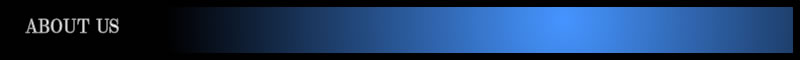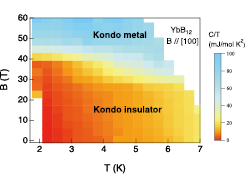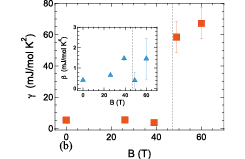# ISSP - The institute for Solid State PhysicsFont Size: (S) / (M) / (L)

- Activity Report 2018 -

F. Iga, Y. H. Matsuda, and Y. Kohama

Magnetic-Field-Induced Kondo Metal State in the Kondo Insulator YbB12Fig. 1. The plot of the Cp / T in the B-T plain with a color gradient scale. The Kondo metal phase appears in high magnetic fields.Fig. 2. Sommerfeld coefficient γ is plotted as a function of the magnetic field. The jump of γ to a large value is a direct evidence of appearance of a Kondo metal with heavy mass quasi particles.

The Kondo effect is one of the most intensively studied many-particle correlation effects and induces intriguing phenomena such as heavy fermions and characteristic behaviors of electrons in quantum dots. There are rare materials in which insulating phases appear with development of the Kondo effect at low temperatures and they are termed Kondo insulator. Since most of the compounds with significant Kondo effect are metallic, the origin of formation of the charge gap has been attracting attention for a long time. Application of magnetic field (B) is expected to control the electronic state of the Kondo insulator through the Zeeman effect, and the magnetic field induced insulator-metal (IM) transition actually occurs in the prototypical Kondo insulator YbB12 at around 50 T . The properties of the high-field metallic phase have never been well understood because the magnetic field required is rather high, which makes microscopic measurements difficult to perform.

Here we report on specific heat (Cp) measurements of YbB12 in high magnetic fields of up to 60 T. The techniques for measurements of specific heat in a pulsed magnetic field have been developed recently  and applied to researches of various kinds of materials. We found that the specific heat sharply increases as the IM transition takes place around 50 T. This largely enhanced value of specific heat coefficient (Cp /T) strongly indicates that a large density of state (DOS) emerges at the Fermi energy and the electronic state corresponds to the Kondo metal state with heavy mass quasi particles.

Figure 1 shows a color plot image of the Cp /T in the B-T plane. The Kondo metal state is found to appear in higher field region. Using the relation Cp /T = γ + βT2, where the second term is due to phonon contribution with a constant β, the Sommerfeld coefficient γ is deduced from the temperature variation of Cp. The deduced γ is plotted as a function of the magnetic field in Fig. 2. A similar plot for β is shown in the inset. The vertical dashed lines denote the magnetic field where the IM transition takes place . A distinct increase of γ occurs along with the IM transition, and the γ at a high-field metal phase is 58 mJ/(mol K2) and 67 mJ/(mol K2) at 49 T and 60 T, respectively. Although β may also have finite magnetic field dependence, it is relatively smaller than the large field-induced change in γ and can be ignored at low temperatures. It is also interesting to note that the obtained γ is as large as that in Kondo metals that exhibit strong valence fluctuations. For instance, γ is 50 mJ/(mol K2) in YbAl3 and 130 mJ/(mol K2) in α-YbAlB4. YbAl3 is one of the typical valence fluctuation compounds, and α-YbAlB4 is the anisotropic heavy fermion compounds with significant valence fluctuations. From the value of γ, it is clearly concluded that the field-induced metal state in YbB12 is regarded as a Kondo metal in the valence fluctuation regime .

We can evaluate the Kondo temperature TK in the high-field Kondo metal phase. The entropy S in the field-induced metal can be estimated from the degeneracy W of the magnetic state of 4f electrons in trivalent Yb ions. The ground state of the Yb3+ ions in the cubic crystal field in YbB12 is Γ8 and the W is 4. The excited Γ6 and Γ7 doublets are almost degenerated and located at a higher energy than Γ8 by about 23 meV. Since the energy gap (15 meV) collapses due to the Zeeman effect at the transition magnetic field and this energy scale is comparable to the excitation energy of the crystal field splitting (23 meV), the three Γ6,7,8 states more or less mix each other in high magnetic fields, and thus, the upper limit of W is 8. The S is safely evaluated to be between R ln4 and R ln8, where R is the gas constant. Using the relation γTK ~ S, TK is deduced to be 172K (for W = 4) and 258 K (for W = 8) at 60 T. The obtained TK is comparable to that of α-YbAlB4 (TK ~ 200 K).

The theoretical work using dynamical mean field theory  predicts the appearance of the Kondo metal phase in high magnetic fields, whose Zeeman energy becomes comparable to the energy gap. According to this calculation, a sharp peak of DOS appears at the IM transition, and the calculated magnetization shows a steep increase at the transition that is in good agreement with the experimentally obtained results. The sharp peak can be considered as the field-induced Kondo resonance peak. In even greater magnetic fields, the Kondo effect is expected to be sufficiently suppressed. The recent report on the magnetization process in YbB12 suggests that the magnetic field for the Kondo breakdown is as high as 120 T .

References

•  K. Sugiyama, F. Iga, M. Kasaya, T. Kasuya, and M. Date, J. Phys. Soc. Jpn. 57, 3946 (1988).
•  Y. Kohama, Y. Hashimoto, S. Katsumoto, M. Tokunaga, and K. Kindo, Meas. Sci. Technol. 24, 115005 (2013).
•  F. Iga, K. Suga, K. Takeda, S. Michimura, K. Murakami, T. Takabatake, and K. Kindo, J. Phys. Conf. Ser. 200, 012064 (2010).
•  T. T. Terashima, Y. H. Matsuda, Y. Kohama, A. Ikeda, A. Kondo, K. Kindo, and F. Iga, Phys. Rev. Lett. 120, 257206 (2018).
•  T. Ohashi, A. Koga, S.-i. Suga, and N. Kawakami, Phys. Rev. B 70, 245104 (2004).
•  T. T. Terashima, A. Ikeda, Y. H. Matsuda, A. Kondo, K. Kindo, and F. Iga, J. Phys. Soc. Jpn. 86, 054710 (2017).

Authers

• T. T. Terashima, Y. H. Matsuda, Y. Kohama, A. Ikeda, A. Kondo, K. Kindo, and F. Igaa
• aIbaraki University